## Friday, October 23, 2009

### Excel Formulas : Countif Multiple Criteria

Many times we need to perform a count based on multiple criteria.

This is very simple if you are using Excel 2007. You have standard excel function Countifs which lets you do the count based on multiple criteria.But if you are using Excel 2003 this becomes tricky as you do not have any standard function that lets you do this. However you can do it using Pivot Tables. Sometimes you just need the count based on multiple criteria to be put into a report and using Pivot tables for such report may not be a viable option.

In such scenarios you need to go for a workaround using excel formula. Considering you have a data table like this and you want to know the number of customers in North Area serviced by Sales Man by name of Ram.

If you have Excel 2007 then it very simple just use
=COUNTIFS(\$B\$13:\$B\$28,"North",\$C\$13:\$C\$28,"Ram") and it will give you count result 3.

For Excel 2003 users My favorite for such kind of calculation is Sumproduct function and you can do it with the help of following formula.
=SUMPRODUCT((\$B\$13:\$B\$28="North")*(\$C\$13:\$C\$28="Ram"))
This formula will give you result as 3 customers.
You can add as many conditions here but be sure that the height of the range is same for all ranges mentioned in this formula

This actually is a workaround for another method array formula.
=SUM((\$B\$13:\$B\$28="North")*(\$C\$13:\$C\$28="Ram")) confirmed with CTRL+Shift+Enter
Once you confirm this formula with CTRL+Shift+Enter it will add {} to the formula which will be visible in the formula bar only.

Look at the screen cast below to know the difference it make to the normal formula once confirmed as CSE formula. Look for the addtional {} added to formula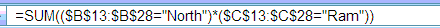You may find it difficult to enter array formula that is why I suggest you to go for SUMPRODUCT method.

### Introducing array formulas in Excel

.

1.I have a column of invoice numbers in an Excel 2003 file. In the next column, I want to do something like a VLOOKUP to see if there is a PDF file of the same name as the invoice number in a certain directory on the network. Can you do a comparison of a text from an Excel cell to names of PDF files.

2.Hello John

Welcome to my blog...

Vlookup will be possible if you have file names in also in your excel file. So you will need to get the list of file names from your file directory on the network. Excel macro for getting file name is given below

Sub ListFiles()
F = Dir("C:\filepath\*.PDF")
Do While Len(F) > 0
ActiveCell.Formula = F
ActiveCell.Offset(1, 0).Select
F = Dir()
Loop
End Sub

You will need to change C:\filepath to your actual path on network. On running this macro you will get the list of PDF file names in excel.

Now I hope doing a Vlookup will be easy.

Thanks.

3.I have a spreadsheet which is attempting to count multiple criteria on another tab within the same spreadsheet.

If the name of the spreadsheet being referenced is "Test", how does that change the COUNTIF formula for 2003 and 2007?

4.Hello Paymon

Welcome to my blog. Considering the same data as in blog post above. But this time data in on sheet named "Test". The formula in this case will be
=SUMPRODUCT((Test!\$B\$13:\$B\$28="North")*(Test!\$C\$13:\$C\$28="Ram"))

This formula will work in Excel 2003 and 2007 both.

However if you have Excel2007 than you can use following formula also

=COUNTIFS(Test!\$B\$13:\$B\$28,"North",Test!\$C\$13:\$C\$28,"Ram")

Thanks
Yogesh Gupta

1.alot of thanks yogesh

5.hi yogesh, plz help me in this..
Names
raam
raam
raam
shaam,raam
shaam,raam
raam

now i need count of rows containing raam i.e.6
and count of rows containing shaam i.e.2

6.@karandeep,

You can do this with the COUNTIF function...

=COUNTIF(\$A\$1:\$D\$100,"*raam*")
=COUNTIF(\$A\$1:\$D\$100,"*shaam*")

Just change the range as needed (I used absolute references in case you planned to copy the formul). Oh, and notice the use of the asterisks... they are wildcards standing for zero or more characters at their location within the text. If you want to put the names in cell rather than hard coding them into the text, you would concatenate the cell with the asterisks (let's assume the name is in E1)...

=COUNTIF(\$A\$1:\$D\$100,"*"&E1&"*")

7.Mr. Gupta,
THANK YOU.
I am bookmarking your site and will be back often.
Sincerely,
Chip Dempsey

8.@Rick - Thanks for your response to Karandeep

Regards//Yogesh Gupta

9.Thank you for having this page! I have been struggling with the task of counting instances of words with multiple criteria. This post really made it clear what I need to do! Now I'm done with my task right quick!
~Nicolle

10.Hi Yogesh, can u pls tell me on how to count multiple opbects in a cell?

11.Hi Yogesh,
Kindly help me with the formula for the following:
I'm using the following formula to calculate time =SUMIF(H21:H266,AG2,I21:I266) where AG2 is a parameter. To count the no. of times a parameter occurs using =COUNTIF(H21:H266,AG2).

But the problem is this calculation is based on single unit, individuals filling the sheet are required to select the parameter everytime if there is a repeatition.

Is there any formula where we can specify numbers 1-50 (dropdown) for units and they can just select the parameter put across the no. of units for it.

Kindly let me know your contact details for further discussion.

Thanks & Regards,

Gauri R.

12.Hi Yogesh

Many Thanks
Shabry

13.Hi Yogesh,

Sorry its SUMPRODUCT, not SUBPRODUCT

14.Hi Shabry

This formula works with all type of data. Let me know how are you using it. It will be better if you can send your file to me along with formula you have used. Pls send file to

yogesh at yogeshguptaonline dot com

Regards//Yogesh Gupta

1.days name status
1 tom done
2 tom pending
3 tom done
4 john pending

Hi yogesh, i need two formula.
1.i need to count how many pending with john
2.and how many pending with john for morethan 2 days.

15.Hi Yogesh,

I'll send the file by email.

Thanks
Shabry

16.Hi Yogesh,

I have created a spreadsheet with four Columns where i have Task that has a drop down(Training,Productivity,Break and so on.)Start Time, End time, Total time spent.

Now in a cell i want to calculate total number of hrs spent on particular task.

Could you suggest me on this..

Regards
Ganesh

17.Hi Ganesh

You can use sumif formula using task and total time spent column. Send me your file in case you need help with the formula.

Regards//Yogesh Gupta

18.How could i get this to work

2 columns
A B
"First Name", "Last name"
John Smith
John Carry
Bob Laurance
John Apple

Now I want to use countifs to get a count of all John's in column A but only if column B is Smith "or" Apple?

Thanks

19.@Yossi,

Give this formula a try...

=SUMPRODUCT((A1:A100="John")*((B1:B100="Smith")+(B1:B100="Apple")))

Of course, you can put the names in cells and then use cell references instead of hard coded names in the formula if you want.

Rick Rothstein (MVP - Excel)

20.This comment has been removed by the author.

21.Hi Yogesh,
I'm having a little trouble with my formula, and I have tried to use your Sumproduct method. I have Excel 2003.

Here it is:
=SUMPRODUCT(('\\filename\file\file1\Inspections\Inspection LOG\[Master Inspection LOG.xls]NBT'!\$B:\$B="Site Visit")*('\\filename\file\file1\Inspections\Inspection LOG\[Master Inspection LOG.xls]NBT'!\$D:\$D,"<="&DATE(2010,7,9)))
I'm looking for "Site Visit" in column B, and want the inspection date to be earlier than July 9, 2010.

Thanks!
Ivy

22.Hi Ivy

I suggest that you replace "<="&DATE(2010,7,9) with <=DATE(2010,7,9). I hope the formula will work after that.

Since dates are numeric numbers you do not require to convert them into string/text for comparision purpose.

Regards//Yogesh Gupta

23.Hi Yogesh,

Hope Yogesh hope you can help with this. I've tried using Sum with Vlook-up but didn't help.

I want to do conditional sum on the value based in column A. For example I've got 4 columns; Col A with name and Col B to Col D with number values. For all values corresponding to Name "A" in Col A i want to sum numbers in Col B to Col D. Can you pls help me with this. Pls see example below if that provides any further clarity:

Name Value 1 Value 2 Value 3
A 8 8 8
A 2 7 3
A 4 9 1
B 4 8 7
B 3 7 2
C 1 7 4
A 2 1 7
B 2 3 6
B 4 6 4
C 5 4 9
C 3 9 8

24.@Amita,

Are you looking for a **single** formula that gives the grand total of the cells in B:D for each row in which Column A cells contain the letter "A"? If so, try this formula...

=SUMPRODUCT((A1:A100="A")*(B1:B100+C1:C100+D1:D100))

Rick Rothstein (MVP - Excel)

25.Hi Yogesh,

I was looking for the "countif multiple options" and stumbled upon your blog after going through several other solutions and this is where I stopped.

Thank you for sharing.

Martin

26.Hi Yogesh,
Great site! I have Excel 2003. I want to match dollar items in one column that are same amt where one is a credit and one is a debit (like balancing a checkbook). I am using this formula to find matches =COUNTIF(\$C\$2:\$C\$1200,C2)and it is working great to find matching dollar amts in Column C. However, I want to add one more criteria. Column D is either Debit or Credit. I only want to match Debits with Credits. Currently, if I have 100.00 in C2 and 100.00 in C3, it will count as 2, regardless of what is in Column D2 and D3. I would like a formula to count only if D2 and D3 are NOT equal. So if C2 is 100.00 and C3 is 100.00 and D2 is Debit and D3 is Credit, that would be a match. Or D2 is a Credit and D3 is a Debit, also a match. But if D2 and D3 are both Debits, that is not a match. If D2 and D3 are both Credits, not a match. Can you please help? Thank you.

27.Dear Yogesh.

I have a spreadhseet and i am using multiple sheets. I am using one sheet as a database, client information, name address etc, and another sheet to capture contact/activity information.

I have already created the formual
=COUNTIF('Mary Contact'!\$C\$2:\$C\$3000,'Basic Database'!C3)to count how many times a client is contacted (data inputted on one sheet to be counted on the main database)the formula recognises the client reference and counts how may times it is inputted on the second sheet.

I now want to create another formula (in a different column) which does the same as the above FIRST and then count if another if column states DNA. Meaning it checks for the client ref then counts the DNA against that ref.

I really hope you can help with this. I have tried different variations had no luck.

I hope this meakes sense!?

Many thanks

28.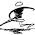I have a spreadsheet with almost 103,000 rows and 28 columns of data from which I'm creating multiple pivot tables.

The rows in Column 'A' contain four values, "Big", "Sky", "Lake", "Lodge". Column 'B' contains a Customer Number, a six-digit code. Column 'C' contains the formula =COUNTIF(\$B\$2:\$B\$10300,\$B2), giving me a count each Customer Number. This is great, I now know if that Customer Number is a one-time or repeat customer.

The problem is, I now need to know if that Customer Number is a repeat customer of "Big", "Sky", "Lake", or "Lodge". In other words, I need to know if they were a customer of at least two of those four.

This formula does not work:

=PRODUCT(COUNTIFS(A\$2:A\$10300,{"Big","Sky","Lake","Lodge"},B\$2:B\$10300,B2))>0

Is there a formula that can return something simple as "True" or "False" - anything that will allow me to pull/sort in a pivot table - for this criteria.

Make sense?
Any/all help is appreciated...

29.Hi Yogesh

Is it possible to use to conditions in the Sumproduct formula you have mentioned? ie
=SUMPRODUCT((\$B\$13:\$B\$28="North OR South")*(\$C\$13:\$C\$28="Ram"))

If Ram exist for two regions (North and South) I want to return the value. I used "OR" here, I know this is not correct. Can you please help me with the correct syntax.

Thanks
Ram.M

30.Hi Yogesh,

I have three columns I need to count:
Column A: "Player"
Column B: "Play:
column C: "Yards"

The first two are simply counts based on criteria.
The A column will be a player's number.
The B column will be "r" for run, or "p" for pass or "x" for nothing attributed to the player
The C column will be the sum of the first two criteria in the "yards" column.

Thanks,

31.Hi Yogesh,

How to use un-need symbol/formula ; if we want to exclude some criteria while using countifs.

32.sometime when i export my data from my software in excel then i insert subtotal for date wise total..bt excel cant show summary of subtotal. i have most important of summary of data for work..so plz. say abt this prblm.

33.HI I NEED TO COMPARE ALTERNATE CELLS WITH COUNTIF PLS HELP

EXAMPLE with column : A5 , C5, E5 or rows : c3,c5,c7,c9

COUNTIF SHOULD NOT INCLUDES B5, D5 & F5 or c2,c4,c6 ETC..

34.Hi! I need to count with 3 different conditions. All data is in one sheet and I need to count the numbers of cases assigned to Person A (condition 1), type of cases assigned to Person A (condition 2) within a month (condition 3).

Greatly appreciate if you can help with this.

Thanks much!

35.Hi,

I need to count the number of Pass and number of Fail for a given date for the list below:

Pass 12/12/2011
Pass 12/13/2011
Fail 12/12/2011
Fail 12/13/2011
Pass 12/12/2011
Pass 12/12/2011

36.=COUNTIF(A2:A7,"pass")
=COUNTIF(A2:A7,"fail")

37.Hi,

the formula given by you =COUNTIF(A2:A7,"pass")
=COUNTIF(A2:A7,"fail") only gives the number of pass/fail....how to get the number of pass for a given date ?

Regards,

Sanjay.

38.sir i want 1 to 100 numbers i want to below 40 to 30 give me formula

39.Hi i have a table with 2 columns columns a customer name and column b id customer i want to creat a type that search on column a the name customer and give me in column c a text (the name of my saler)

40.hi yogesh,

Can you help me out by sharing your thoughts about the viability of using range based criterion like '>10and <12' alongwith countif ?

Koushik Ghosh

41.hi i have 3 A,B&C colums with different value & name. i want to count to equal & < value of Colume B to C related of Colume A. example is below for your reference.
Q:count how many Fish (Colum A) value of B is equal & < to C.

A B C
Fish 1 1
Cat 1 1
Fish 2 1
Bird 1 1
Fish 1 1

42.i want to count below columes value with out repeated same value.for example 123 is write in Colum A 3times & 245 write 2 times & 356 only 1 time & so on.... but i want that which value repeated or not. its count 1. & we take total count of said Colum in Last cell.

Colum A
123
123
245
245
123
356

43.This comment has been removed by the author.

44.Thanks a Lot Sir

45.I am Looking for the Output with no of cells filled in the below mentioned formula in 2007 Excel. But need to apply the formula in 2003 Excel. Kindly suggest the way forward.

COUNTIFS(C:C,"3/1/2012",D:D,"<>")

Tried in SUMPRODUCT Formula but not working....

46.Sir

I want to Calculate interest @2% P.M on Late Payment from Customer on Every invoices.Grace Period Should be 30 Days.Invoices Should be adjusted on FIFO Basis with Payment.Can you Help me for this.I will be grateful for this.My E mail id is abhishekp12in@gmail.com

Thanks
Abhishek

47.Dear Yogesh,

I want to counting in two condition for ex. =countif(a1:a5,"2<>3") in between two number but its not working, so please suggest me. my Email ID is personal.mahesh33@gmail.com

48.Dear Yogesh,

I want to counting in two condition for ex. =countif(a1:a5,"2<>3") in between two number but its not working, so please suggest me. my Email ID is personal.mahesh33@gmail.com

49.Dear Yogesh
Col.A (date) Col.B (Plan) Col.C (Code of Salesman)
20111213 149 80001603
20111213 149 80001603
20111130 91 80001709
20111130 165 80001709
20111130 91 80001709
20111130 91 80001709
20111130 165 80001709
20111129 805 80001709
20111129 805 80001709
20111129 805 80001709
20111129 91 80001709
20120131 802 80001725
20120124 91 80001904
20120124 91 80001904
20120124 91 80001904
20120118 91 80001904
20120118 91 80001904
20111031 805 80001904
20111121 805 80001904
20120130 91 80001904
20111202 165 80001904
20110628 179 3449177
20110928 179 3449177
20111031 14 3449177
20110425 165 3868177
20110425 75 3868177

I want to count the number of salesman (excluding duplicates) who sold a particular plan (say 165) between 20110425 to 20111223. Kindly suggest me a formula. My Email ID is ratheeajit.singh@gmail.com

50.I have one I need solving.

I have 4-columns that I want to search for a list of possible matches.

Here's a sample:

Here's the column, I want to search...
A B C D
1 -95A0 -95A0 NONE 11H3C
2 -95A0 -95A0 NONE 11F3Q
3 -95A0 -95A0 NONE 62E3E
4 -90G0 -90G0 NONE

I want to countif (or whatever) everycell above for any cell that has the following...
A B
10 11B 46P
11 11E 46S
12 11F 47S
13 11G 48A

Example: A12 (11F) is found in D2 (11F3Q)--this should count as 1.

1.Addendum: I would like to avoid a long formula. It seems to work well on individual cell criteria [ex: =COUNTIF(BB2:BD586,BD631&"*")], but I would like to do something like this: =COUNTIF(BB2:BD586, BC591&"*":BD631&"*"), which of course doesn't work this way.

51.Hello--
I am desperate for a formula that will find matches when the first 11 digits in a cell equal the last 11 digits in a cell, in the same column. Like this:

Cell A
12345678911445555
78145612345678911

I hope you can help. Thanks!

1.=IF(LEFT(A1;11)=RIGHT(A1;11);"11 char in beginning and end match"; "No match")
You'd like also to keep the cell format as Text, since numbers will convert your long number to exponential presentation and there formula will not work.

52.Hi!

Need help with COUNTIF or COUNTIFS, I have a data where I need to count the no. of entries in a column which are for a particular month, say for March so what should be the formula for this.

Thanks & Regards,
Pritish Kamal

53.Hi!

Need help with COUNTIF or COUNTIFS, I have a data where I need to count the no. of entries in a column which are for a particular month, say for March so what should be the formula for this.

Thanks & Regards,
Pritish Kamal

54.Hello Yogesh, can you help me figure out why I cannot get this to work?
=COUNTIF(I2:R11,">=B2")-COUNTIF(I2:R11,">=C2")

If I enter the formula in this fashion, it works fine, but now when I want to take the value from a cell that can change.
=COUNTIF(I2:R11,">=0")-COUNTIF(I2:R11,">=12")

1.Pls enter it like this to work

=COUNTIF(I2:R11,">="&B2)-COUNTIF(I2:R11,">="&C2)

You need to join the text string with the cell reference rather than just putting it in the text string. The way you have used it excel does not recognized it as cell reference.

55.Hello Yogesh,

Conversion of Numeric to Character in Excel 2003 without writing any code by applying only formula.

Regards,

1.Hi Ram

Not clear about your request, can you give some example of what do you need.

Thanks

56.Small Note: SUMPRODUCT formula will not work for entire column.
Example: =SUMPRODUCT((\$B:\$B="North")*(\$C:\$C="Ram"))
The above formula will give a error.

Instead use a cell range as suggested by Yogesh.
=SUMPRODUCT((\$B\$13:\$B\$28="North")*(\$C\$13:\$C\$28="Ram"))

Thanks.

57.Hello Yogesh,

can you help me...

What is the formula for my situasion:

eg:
A A A A A - 5A (summaries: all A)
A B A A A - 4A 1B (summaries: at least 1B)
A B B B B - 1A 4B (summaries: at least 1B)
A B B A C - 2A 2B 1C (summaries: at least 1C)
B C D B B - 3B 1C 1D (summaries: at least 1D)
E E E E E - 5E (summaries: all E)

What is the formula to count:
all A - 1 person
at least 1B - 2 person
at least 1C - 1 person
at least 1D - 1 person
all E - 1 person

and so on

58.Hi
I have data for 5 Teams - A,B,C,D,E and each team has 10 members. Now I would like to have a formulae to calualate, No of present "P" within each team. No of members who have >7:30, <7:00>7:30 staffed hours ..

Regards
Guru
MIS

59..Thanks Yogesh,

Regards,
Divy

60.Hi Dears

how can I use countifs with three column on of them has two text criteria

i tried this one but it not work
=COUNTIFS(H2:H223,COUNTIF(H2:H223,"RFT")+COUNTIF(H2:H223,"On air"))

61.Hi Dears

how can I use countifs with three column on of them has two text criteria

i tried this one but it is not work:
=COUNTIFS(H2:H223,COUNTIF(H2:H223,"RFT")+COUNTIF(H2:H223,"On air"))

1.Try This

=COUNTIFS(H2:H223,{"RFT","On air"})

62.Hello,

I am using this formula:
=COUNTIF(J31:K31,"*A*")+COUNTIF(J31:K31,"*P*")

I also have PP as a value and it is counting this. I only want to count the P. Help???

1.Use This Formula

=COUNTIFS(Attendance!J31:K31,{"*A*","*P*"})

63.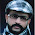Thanks a lot. I was struggling with multiple criteria function for the last 3 days. Since I am using MS Office 2003, I was unable to use the "COUNTIFS" function.
I sought help from here and it really worked for me.
Thanks a lot again.

64.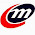DATE RECEIPTNO AMOUNT CASH/CHE/D.D/RTGS
1-Sep-14 AK-290 29,513.00 CHEQUE
1-Sep-14 AK-291 5,000.00 CASH
1-Sep-14 AK-295 2,000.00 CASH
1-Sep-14 AK-296 658,694.00 CHEQUE

HI SIR
SUM FROM DATE WISE SUM (CASH CHE DD RTGS)

65.Fabulous, what a blog it is! This weblog provides helpful data to us, keep it up. itunes login account

66.Really cool post, highly informative and professionally written and I am glad to be a visitor of this perfect blog, thank you for this rare info!
MS office course in chennai

67.Nice and good article. It is very useful for me to learn and understand easily. Thanks for sharing your valuable information and time. Please keep updating.
Web Design Training

68.69.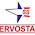Thanks for sharing this information.
Want to Buy Step Down Transformer or Are you Curious about What is Step Down Transformer? Read the blog to get your queries resolved before making a purchase.

70.71.